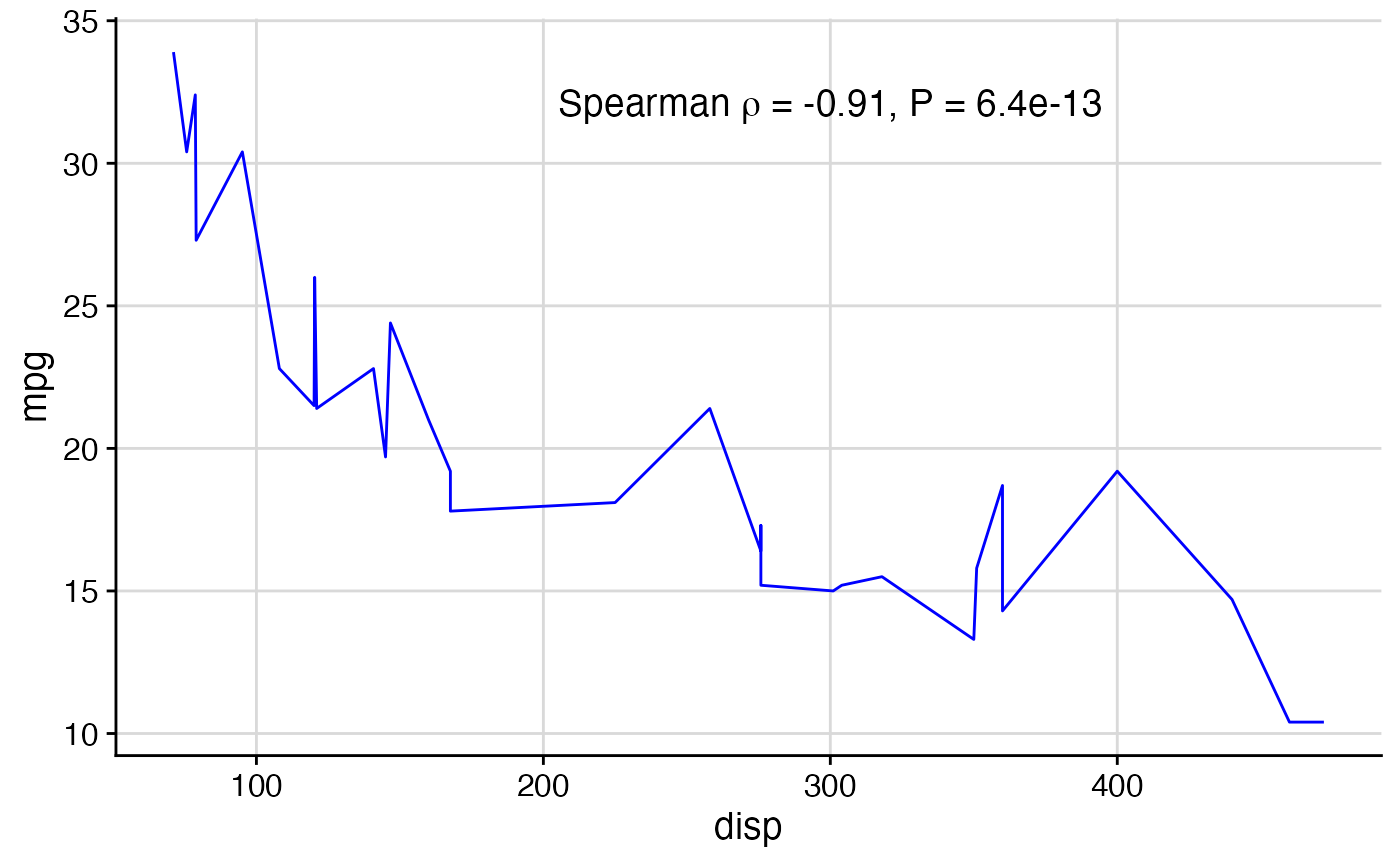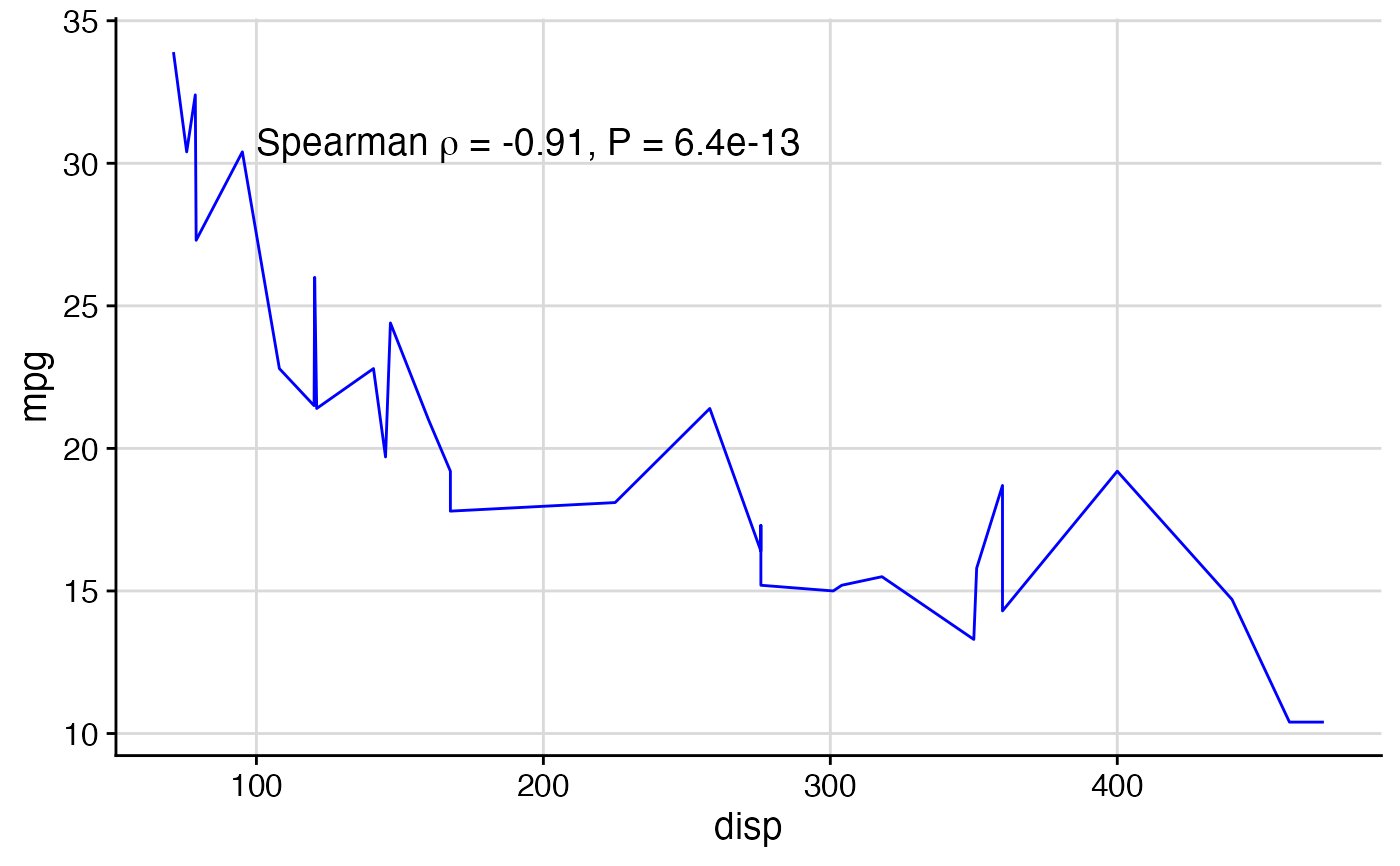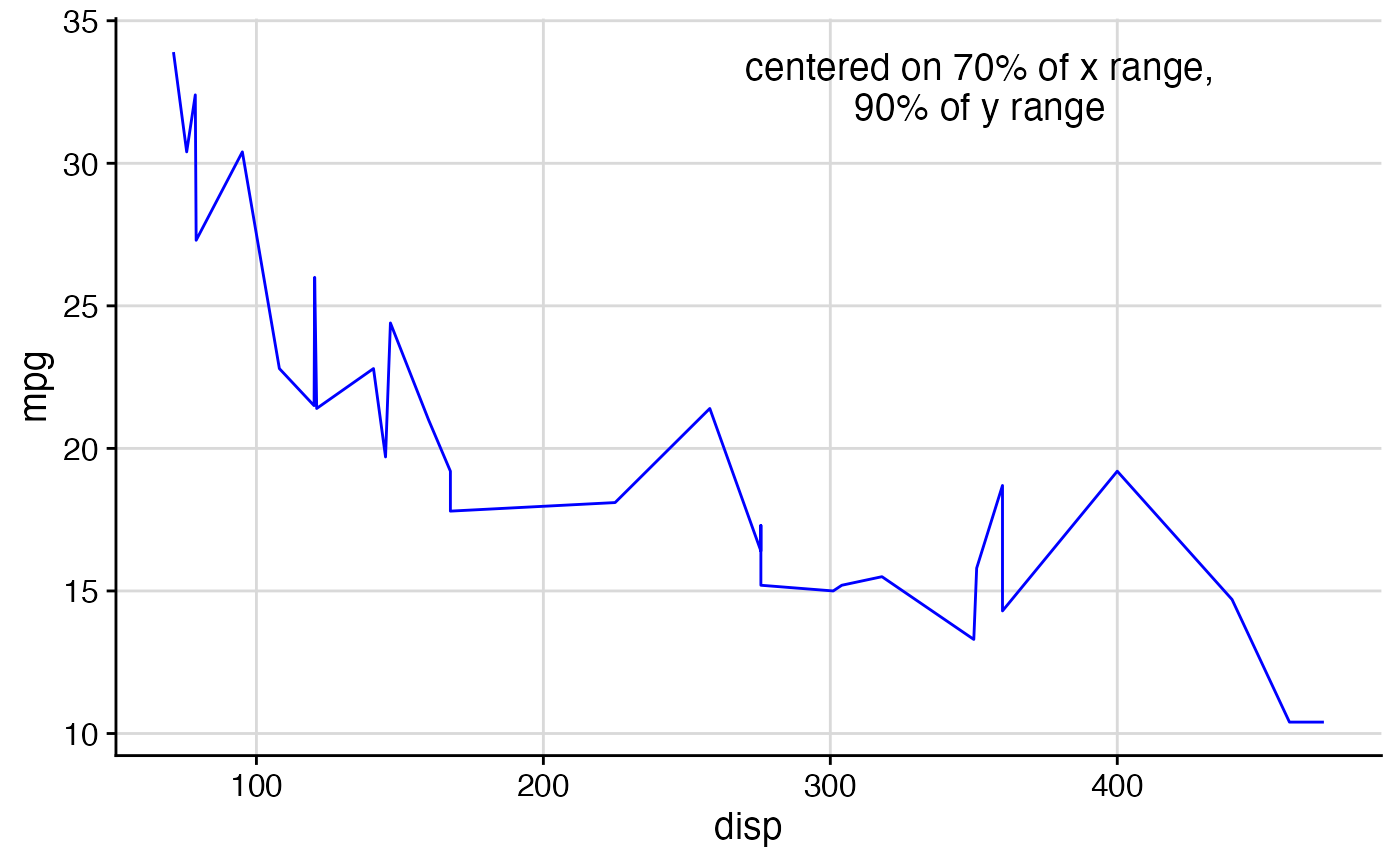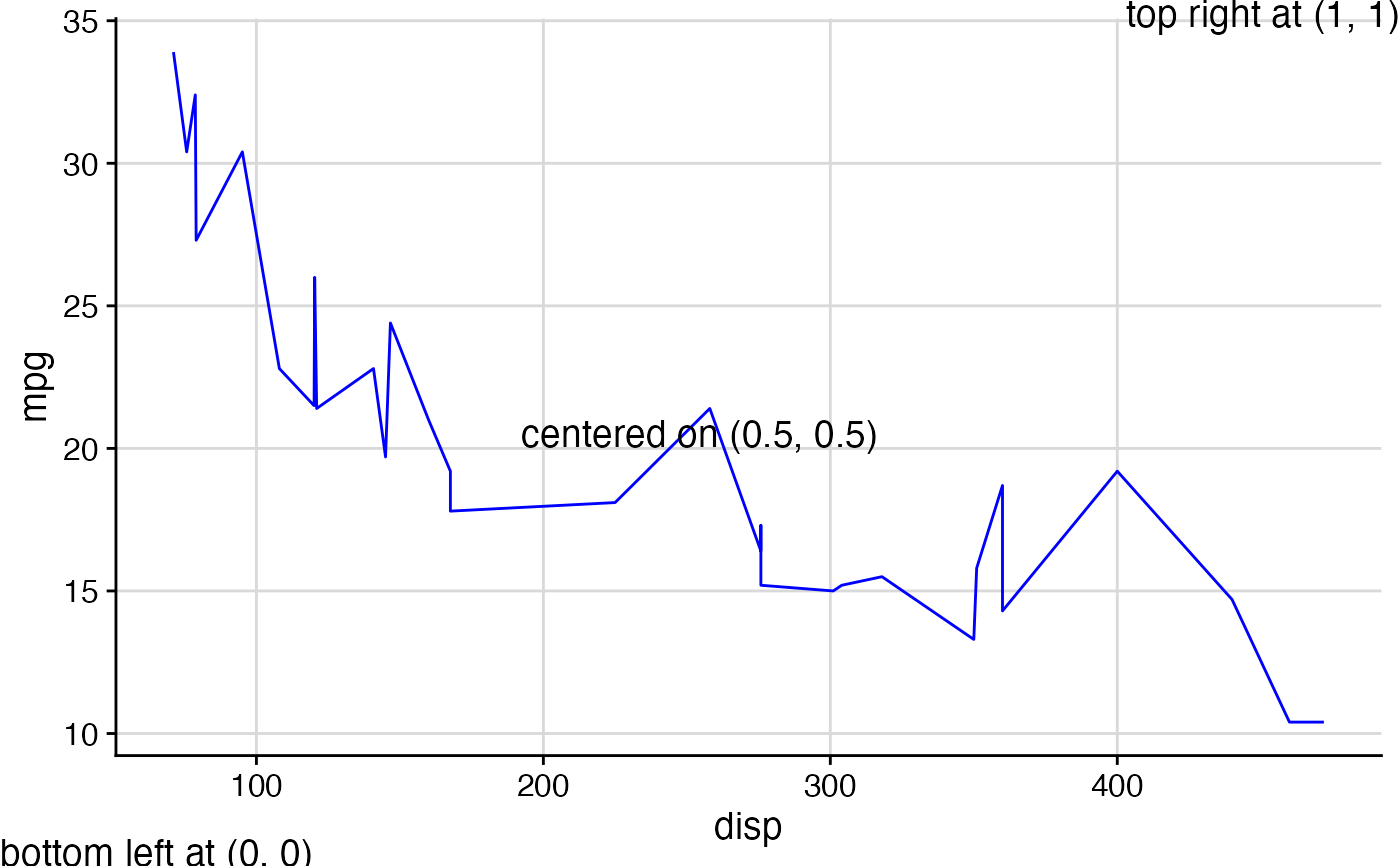This function can draw either a character string or mathematical expression at the given coordinates. It works both on top of ggdraw and directly with ggplot, depending on which coordinate system is desired (see examples).

draw_label(
label,
x = 0.5,
y = 0.5,
hjust = 0.5,
vjust = 0.5,
fontfamily = "",
fontface = "plain",
color = "black",
size = 14,
angle = 0,
lineheight = 0.9,
alpha = 1,
colour
)

Arguments

label String or plotmath expression to be drawn. The x location (origin) of the label. The y location (origin) of the label. Horizontal justification. Default = 0.5 (centered on x). 0 = flush-left at x, 1 = flush-right. Vertical justification. Default = 0.5 (centered on y). 0 = baseline at y, 1 = ascender at y. The font family The font face ("plain", "bold", etc.) Text color Point size of text Angle at which text is drawn Line height of text The alpha value of the text

Details

By default, the x and y coordinates specify the center of the text box. Set hjust = 0, vjust = 0 to specify the lower left corner, and other values of hjust and vjust for any other relative location you want to specify.

library(ggplot2) # setup plot and a label (regression description) p <- ggplot(mtcars, aes(disp, mpg)) + geom_line(color = "blue") + theme_half_open() + background_grid(minor = 'none') out <- cor.test(mtcars$disp, mtcars$mpg, method = 'sp', exact = FALSE) label <- substitute( paste("Spearman ", rho, " = ", estimate, ", P = ", pvalue), list(estimate = signif(out$estimate, 2), pvalue = signif(out$p.value, 2)) ) # Add label to plot, centered on {x,y} (in data coordinates) p + draw_label(label, x = 300, y = 32)